## Thompson scattering

Consider a plane electromagnetic wave of angular frequencyinteracting with a free electron of mass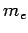and charge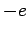. Suppose that the wave is polarized such that its associated electric field is parallel to the-axis: i.e.,(1120)

Recall, from Sect. 4.7, that as long as the electron remains non-relativistic, the force exerted on it by the electromagnetic wave comes predominantly from the associated electric field. Hence, the electron's equation of motion can be written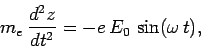(1121)

which can be solved to give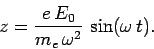(1122)

So, in response to the wave, the electron oscillates backward and forward in the direction of the wave electric field. It follows that the electron can be thought of as a sort of oscillating electric dipole, with dipole moment(1123)

where. (For the moment, let us not worry about the positively charged component of the dipole.) Now, we know that an oscillating electric dipole emits electromagnetic radiation. Hence, it follows that a free electron placed in the path of a plane electromagnetic wave will radiate. To be more exact, the electron scatters electromagnetic radiation from the wave, since the radiation emitted by the electron is not necessarily in the same direction as the wave, and any energy radiated by the electron is ultimately extracted from the wave. This type of scattering is called Thompson scattering.

It follows from Eq. (1119) that the differential power scattered from a plane electromagnetic wave by a free electron into solid angle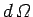takes the form(1124)

Now, the mean energy flux of the incident electromagnetic wave is written(1125)

It is helpful to introduce a quantity called the differential scattering cross-section. This is defined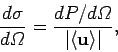(1126)

and has units of area over solid angle. Somewhat figuratively, we can think of the electron as offering a target of area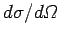to the incident wave. Any wave energy which falls on this target is scattered into the solid angle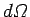. Likewise, we can also define the total scattering cross-section,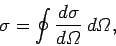(1127)

which has units of area. Again, the electron effectively offers a target of areato the incident wave. Any wave energy which falls on this target is scattered in some direction or other. It follows from Eqs. (1124) and (1125) that the differential scattering cross-section for Thompson scattering is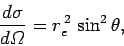(1128)

where the characteristic length(1129)

is called the classical electron radius. An electron effectively acts like it has a spatial extentas far as its iteration with electromagnetic radiation is concerned. As is easily demonstrated, the total Thompson scattering cross-section is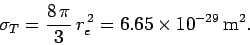(1130)

Note that both the differential and the total Thompson scattering cross-sections are completely independent of the frequency (or wave-length) of the incident radiation.

A scattering cross-section of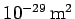does not sound like much. Nevertheless, Thompson scattering is one of the most important types of scattering in the Universe. Consider the Sun. It turns out that the mean mass density of the Sun is similar to that of water: i.e., about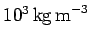. Hence, assuming that the Sun is made up predomintely of ionized hydrogen, the mean number density of electrons in the Sun (which, of course, is the same as the number density of protons) is approximately, where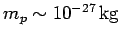is the mass of a proton. Let us consider how far, on average, a photon in the Sun travels before being scattered by free electrons. If we think of an individual photon as sweeping out a cylinder of cross-sectional area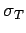, then the photon will travel an average length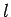, such that a cylinder of areaand lengthcontains about one free electron, before being scattered. Hence,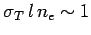, or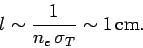(1131)

Given that the radius of the Sun is approximately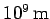, it is clear that solar photons are very strongly scattered by free electrons. In fact, it can easily be demonstrated that it takes a photon emitted in the solar core many thousands of years to fight its way to the surface because of Thompson scattering.

After the Big Bang'', when the Universe was very hot, it consisted predominately of ionized hydrogen (and dark matter), and was consequently opaque to electromagnetic radiation, due to Thompson scattering. However, as the Universe expanded, it also cooled, and eventually became sufficiently cold (when the mean temperature was about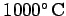) for any free protons and electrons to combine to form molecular hydrogen. It turns out that molecular hydrogen does not scatter radiation anything like as effectively as free electrons (see the next section). Hence, as soon as the Universe became filled with molecular hydrogen, it effectively became transparent to radiation. Indeed, the so-called cosmic microwave background is the remnant of radiation which was last scattered when the Universe was filled with ionized hydrogen (i.e., when it was about). Astronomers can gain a great deal of information about the conditions in the early Universe by studying this radiation.

Incidentally, it is clear from Eqs. (1129) and (1130) that the scattering cross-section of a free particle of charge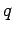and massis proportional to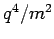. It follows that the scattering of electromagnetic radiation by free electrons is generally very much stronger than the scattering by free protons (assuming that the number densities of both species are similar).